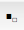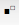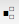# Preface

This tutorial was made solely for the purpose of education and it was designed for students taking Applied Math 0330. It is primarily for students who have very little experience or have never used Mathematica and programming before and would like to learn more of the basics for this computer algebra system. As a friendly reminder, don't forget to clear variables in use and/or the kernel. The Mathematica commands in this tutorial are all written in bold black font, while Mathematica output is in normal font.

Finally, you can copy and paste all commands into your Mathematica notebook, change the parameters, and run them because the tutorial is under the terms of the GNU General Public License (GPL). You, as the user, are free to use the scripts for your needs to learn the Mathematica program, and have the right to distribute this tutorial and refer to this tutorial as long as this tutorial is accredited appropriately. The tutorial accompanies the textbook Applied Differential Equations. The Primary Course by Vladimir Dobrushkin, CRC Press, 2015; http://www.crcpress.com/product/isbn/9781439851043

# Case Sensitivity

In fact, every predefined symbol in Mathematica begins with a capital letter. One advantage of this is that you can define your own symbols beginning with lowercase letters, knowing that you will not conflict with any of Mathematica's names. Mathematica is case sensitive for both variables that can be defined and for commands. The variable a (lower case) is different from A (upper case). You will know if the command that you enter is correct because the color of the command will change to black. The irrational constant Pi (=3.141926...) can be entered either as Pi or, to see Greek letter, as \[Pi] (this is how Mathematica displays Greek letters).
One of the key aspects of using Mathematica is knowing how to enter a command. Once you have typed the command, hold shift down and then hit enter. Hitting shift and enter simultaneously allows you to enter the command, but just hitting enter has no effect.

Subscripts can be very helpful when differentiating between variables. To create a subscript first navigate to your Basic Math Assistant palette. If it is not already up navigate to Palettes in your toolbar and it will be your first choice. On the toolbar scroll down until you see typesetting. SelectIf you wish to add a subscript to a variable that has already been typed, simply highlight the variable before you select.   If you wish to add a superscript, select.   If you wish to add both a subscript and a superscript select.   If you wish to avoid the toolbar you can just as easily type in your subscripts and superscripts using the format shown below

Subscript[x,3]
Superscript[x,a]
Subscript[Superscript[x,a],3]
Out= $$x^a_3$$

Consider the following calculation:

In:= Tan[Pi/3]
Out= Sqrt
Another thing to notice from the previous example is that Mathematica knows that the tangent of $$\pi /3$$ is exactly a square root of 3; it does not return an estimate like 1.73205, as a handheld calculator might.
Now compare the previous example with this:
In:= Tan[Pi/3.0]
Out= 1.73205

The only difference between the two examples is that the integer 3 in the first was replaced by the floating point number 3.0 in the second. This difference was enough to cause Mathematica to return a floating point (approximate) result in the second example.
Here is an important rule: An expression involving only exact (symbolic) quantities will be evaluated exactly (symbolically), while an expression involving one or more floating point numbers will be evaluated approximately using floating point arithmetic.

Use  to explicitly indicate the precision to assume in a number:
In:= 3.141592620
Out= 3.1415926000000000000

There are two more points that I would like to emphasize. First of all, one will often wish to perform a calculation in several steps. Mathematica makes it possible to refer to previous results in a couple of ways. The "%" character always represents the last output. To refer to an earlier output (not just the most recent), use the "Out" keyword, followed by the index of the output. For example, Out always refers to the first output of your Mathematica session:

In:= Out
Out= 5
You can evaluate a symbolic expression in floating point using the N operator:
In:= N[Sqrt]
Out= 1.73205

Any built-in command/function in Mathematica has a literal/string equivalent. This is most easily seen with the help of the built-in function FullForm, which shows the internal representation of any object/expression, in the way it is really "seen" by the kernel. For instance:

In:= FullForm[x*Tan[y + z]]
Out= Times[x, Tan[Plus[y,z]]]
A tree form can be seen using TreeForm command:
In:= TreeForm[x*Tan[y + z]]
Since it is a tree, it is possible to index and access the subexpressions. For instance,
In:= a=TreeForm[x*Tan[y + z]]
In:= a[[1,2]]
Out= Tan[y+z]
In general, an expression outside the square brackets has a name - it is called a head of expression, or just head. There is a built-in function with the same name, which allows one to obtain the head of an arbitrary expression. For example:
Out= TreeForm

Rewrite Rules: A typical rule looks like a -> b where in general < a > and < b > are some Mathematica expressions. The rule just says: whenever < a > is encountered, replace it by < b >. A pattern is essentially any expression with some part of it replaced by "blank" (Blank[]), which is a placeholder for any expression - that is, instead of that part there can be anything (this is somewhat oversimplified). The literal equivalent for Blank[] is the single underscore ("_") symbol. For instance, f[x_] means f[anything].

The application of rules is left - associative, meaning that in the expression < expr /. rule1 /. rule2 > is legitimate, and first the rule (or rules if this is a list of rules, see below) < rule1 > will be applied to < expr >, and then the rule (s) < rule2 > will be applied to the result. It is very important to remember that the rules are local. This means that when the rule rewrites an object to which it applies into something else, it changes the copy of it, while the initial object remains unchanged.

Example: In this example, we first start with the Clear command to remove previous value f. Realistically you will only be using the command as ClearAll is more often used in more advanced computations than will be covered in this tutorial.

In:= Clear[f]
In:= f[x_]= x^2 - Sin[x]
In:= f[Sqrt], f[Oliver]
Out= {9 - Sin, Oliver^2 - Sin[Oliver]}
■
To see the internal form of this rule - how it is stored in the rule base - one can use the built-in DownValues command. With its help we see:
In:= DownValues[f]
Out= {HoldPattern[f[x_]] :-> x^2 - Sin[x]}
As another starting example, let us define a function which is linear on quadratic numbers, cubic on odd numbers and is a tangent function for all other inputs:
Clear[f] f[x_ ?EvenQ] := x^2;
f[x_ ?OddQ] := x^3;
f[x_] := Tan[Pi*x]
{f, f, f, f, f[3/2], f[Oliver], f[Pi]}
{1, 4, 27, 16, ComplexInfinity, Tan[Oliver \[Pi]], Tan[\[Pi]^2]}
Any name which has a head Symbol and is not a name used by the system, is legal to associate some rules (global definitions) with - in other words, a legal name for a variable or a function. It can not start with a number, but can contain numbers. It can contain both capital and small letters, and Mathematica is case-sensitive, so names like AA and Aa are considered different. There are several special symbols that should not be used in symbol names, such as @,#,\$,%,^,&,*,!,~,. What may be surprising for C programmers is that the underscore <_> should not be used either - it has a different purpose in Mathematica.

If you are in doubt whether your symbol is legitimate, you can always use the Head[Unevaluated[yoursymbol]] command to check its head: if it is, you are in business. The reasons why you need Unevaluated` are a bit involved to discuss now, but basically it guarantees that the Head function tests the head of your original symbol rather than what it may evaluate to.